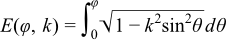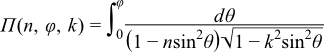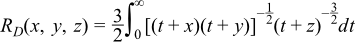#Boost C++ Libraries

...one of the most highly regarded and expertly designed C++ library projects in the world.

This is the documentation for an old version of boost. Click here for the latest Boost documentation.

#### Elliptic Integral Overview

The main reference for the elliptic integrals is:

M. Abramowitz and I. A. Stegun (Eds.) (1964) Handbook of Mathematical Functions with Formulas, Graphs, and Mathematical Tables, National Bureau of Standards Applied Mathematics Series, U.S. Government Printing Office, Washington, D.C.

Mathworld also contain a lot of useful background information:

##### Notation

All variables are real numbers unless otherwise noted.

##### Definitionis called elliptic integral if R(t, s) is a rational function of t and s, and s2 is a cubic or quartic polynomial in t.

Elliptic integrals generally can not be expressed in terms of elementary functions. However, Legendre showed that all elliptic integrals can be reduced to the following three canonical forms:

Elliptic Integral of the First Kind (Legendre form)Elliptic Integral of the Second Kind (Legendre form)Elliptic Integral of the Third Kind (Legendre form)whereNoteφ is called the amplitude. k is called the modulus. α is called the modular angle. n is called the characteristic.
CautionPerhaps more than any other special functions the elliptic integrals are expressed in a variety of different ways. In particular, the final parameter k (the modulus) may be expressed using a modular angle α, or a parameter m. These are related by: k = sinα m = k2 = sin2α So that the integral of the third kind (for example) may be expressed as either: Π(n, φ, k) Π(n, φ \ α) Π(n, φ| m) To further complicate matters, some texts refer to the complement of the parameter m, or 1 - m, where: 1 - m = 1 - k2 = cos2α This implementation uses k throughout: this matches the requirements of the Technical Report on C++ Library Extensions. However, you should be extra careful when using these functions!

When φ = π / 2, the elliptic integrals are called complete.

Complete Elliptic Integral of the First Kind (Legendre form)Complete Elliptic Integral of the Second Kind (Legendre form)Complete Elliptic Integral of the Third Kind (Legendre form)Carlson [Carlson77] [Carlson78] gives an alternative definition of elliptic integral's canonical forms:

Carlson's Elliptic Integral of the First Kindwhere x, y, z are nonnegative and at most one of them may be zero.

Carlson's Elliptic Integral of the Second Kindwhere x, y are nonnegative, at most one of them may be zero, and z must be positive.

Carlson's Elliptic Integral of the Third Kindwhere x, y, z are nonnegative, at most one of them may be zero, and p must be nonzero.

Carlson's Degenerate Elliptic Integralwhere x is nonnegative and y is nonzero.

NoteRC(x, y) = RF(x, y, y) RD(x, y, z) = RJ(x, y, z, z)
##### Duplication Theorem

Carlson proved in [Carlson78] that##### Carlson's Formulas

The Legendre form and Carlson form of elliptic integrals are related by equations:In particular,##### Numerical Algorithms

The conventional methods for computing elliptic integrals are Gauss and Landen transformations, which converge quadratically and work well for elliptic integrals of the first and second kinds. Unfortunately they suffer from loss of significant digits for the third kind. Carlson's algorithm [Carlson79] [Carlson78], by contrast, provides a unified method for all three kinds of elliptic integrals with satisfactory precisions.

##### References

Special mention goes to:

A. M. Legendre, Traitd des Fonctions Elliptiques et des Integrales Euleriennes, Vol. 1. Paris (1825).

However the main references are:

1. M. Abramowitz and I. A. Stegun (Eds.) (1964) Handbook of Mathematical Functions with Formulas, Graphs, and Mathematical Tables, National Bureau of Standards Applied Mathematics Series, U.S. Government Printing Office, Washington, D.C.
2. B.C. Carlson, Computing elliptic integrals by duplication, Numerische Mathematik, vol 33, 1 (1979).
3. B.C. Carlson, Elliptic Integrals of the First Kind, SIAM Journal on Mathematical Analysis, vol 8, 231 (1977).
4. B.C. Carlson, Short Proofs of Three Theorems on Elliptic Integrals, SIAM Journal on Mathematical Analysis, vol 9, 524 (1978).
5. B.C. Carlson and E.M. Notis, ALGORITHM 577: Algorithms for Incomplete Elliptic Integrals, ACM Transactions on Mathematmal Software, vol 7, 398 (1981).
6. B. C. Carlson, On computing elliptic integrals and functions. J. Math. and Phys., 44 (1965), pp. 36-51.
7. B. C. Carlson, A table of elliptic integrals of the second kind. Math. Comp., 49 (1987), pp. 595-606. (Supplement, ibid., pp. S13-S17.)
8. B. C. Carlson, A table of elliptic integrals of the third kind. Math. Comp., 51 (1988), pp. 267-280. (Supplement, ibid., pp. S1-S5.)
9. B. C. Carlson, A table of elliptic integrals: cubic cases. Math. Comp., 53 (1989), pp. 327-333.
10. B. C. Carlson, A table of elliptic integrals: one quadratic factor. Math. Comp., 56 (1991), pp. 267-280.
11. B. C. Carlson, A table of elliptic integrals: two quadratic factors. Math. Comp., 59 (1992), pp. 165-180.
12. B. C. Carlson, Numerical computation of real or complex elliptic integrals. Numerical Algorithms, Volume 10, Number 1 / March, 1995, p13-26.
13. B. C. Carlson and John L. Gustafson, Asymptotic Approximations for Symmetric Elliptic Integrals, SIAM Journal on Mathematical Analysis, Volume 25, Issue 2 (March 1994), 288-303.

The following references, while not directly relevent to our implementation, may also be of interest:

1. R. Burlisch, Numerical Compuation of Elliptic Integrals and Elliptic Functions. Numerical Mathematik 7, 78-90.
2. R. Burlisch, An extension of the Bartky Transformation to Incomplete Elliptic Integrals of the Third Kind. Numerical Mathematik 13, 266-284.
3. R. Burlisch, Numerical Compuation of Elliptic Integrals and Elliptic Functions. III. Numerical Mathematik 13, 305-315.
4. T. Fukushima and H. Ishizaki, Numerical Computation of Incomplete Elliptic Integrals of a General Form. Celestial Mechanics and Dynamical Astronomy, Volume 59, Number 3 / July, 1994, 237-251.